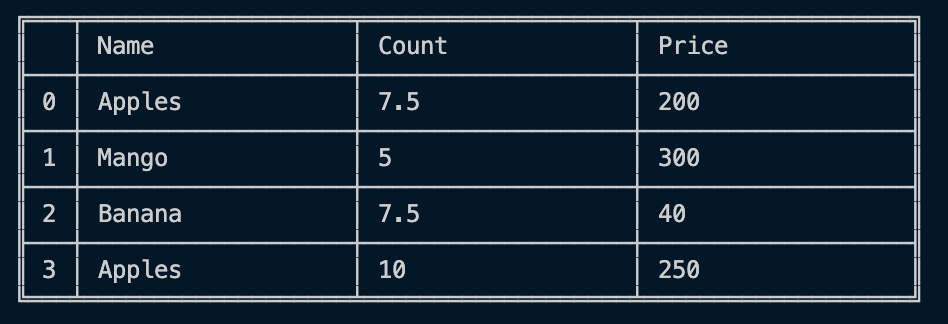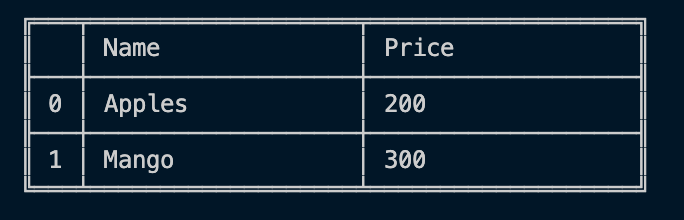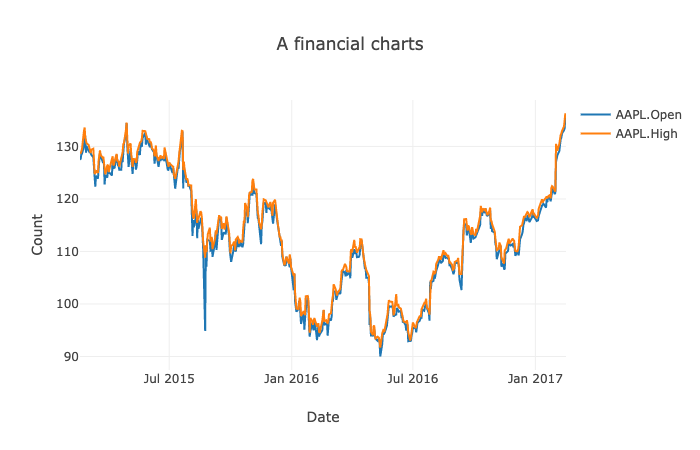Introducing Danfo.js, a Pandas-like Library in JavaScript
আগস্ট ২৫, ২০২০
A guest post by Rising Odegua, Independent Researcher; Stephen Oni, Data Science Nigeria

Danfo.js is an open-source JavaScript library that provides high-performance, intuitive, and easy-to-use data structures for manipulating and processing structured data. Danfo.js is heavily inspired by the Python Pandas library and provides a similar interface/API. This means that users familiar with the Pandas API and know JavaScript can easily pick it up.One of the main goals of Danfo.js is to bring data processing, machine learning and AI tools to JavaScript developers. This is in line with our vision and essentially the vision of the TensorFlow.js team, which is to bring ML to the web. Open-source libraries like Numpy and Pandas revolutionise the ease of manipulating data in Python and lots of tools were built around them, thus driving the bubbling ecosystem of ML in Python.

Danfo.js is built on TensorFlow.js. That is, as Numpy powers Pandas arithmetic operations, we leverage TensorFlow.js to power our low-level arithmetic operations.

### Some of the main features of Danfo.js

Danfo.js is fast. It is built on TensorFlow.js, and supports tensors out of the box. This means you can load Tensors in Danfo and also convert Danfo data structure to Tensors. Leveraging these two libraries, you have a data processing library on one hand (Danfo.js), and a powerful ML library on the other hand (TensorFlow.js).

In the example below, we show you how to create a Danfo DataFrame from a tensor object:
``````const dfd = require("danfojs-node")
const tf = require("@tensorflow/tfjs-node")

let data = tf.tensor2d([[20,30,40], [23,90, 28]])
let df = new dfd.DataFrame(data)
let tf_tensor = df.tensor
console.log(tf_tensor);
tf_tensor.print()``````
Output:
``````Tensor {
kept: false,
isDisposedInternal: false,
shape: [ 2, 3 ],
dtype: 'float32',
size: 6,
strides: [ 3 ],
dataId: {},
id: 3,
rankType: '2'
}
Tensor
[[20, 30, 40],
[23, 90, 28]]
``````
You can easily convert Arrays, JSONs, or Objects to DataFrame objects for manipulation.

JSON object to DataFrame:
``````const dfd = require("danfojs-node")
json_data = [{ A: 0.4612, B: 4.28283, C: -1.509, D: -1.1352 },
{ A: 0.5112, B: -0.22863, C: -3.39059, D: 1.1632 },
{ A: 0.6911, B: -0.82863, C: -1.5059, D: 2.1352 },
{ A: 0.4692, B: -1.28863, C: 4.5059, D: 4.1632 }]
df = new dfd.DataFrame(json_data)
df.print()``````
Output:Object array with column labels to DataFrame:
``````const dfd = require("danfojs-node")
obj_data = {'A': [“A1”, “A2”, “A3”, “A4”],
'B': ["bval1", "bval2", "bval3", "bval4"],
'C': [10, 20, 30, 40],
'D': [1.2, 3.45, 60.1, 45],
'E': ["test", "train", "test", "train"]
}
df = new dfd.DataFrame(obj_data)
df.print()``````
Output:You can easily handle missing data (represented as NaN) in floating point as well as non-floating point data:
``````const dfd = require("danfojs-node")
let data = {"Name":["Apples", "Mango", "Banana", undefined],
"Count": [NaN, 5, NaN, 10],
"Price": [200, 300, 40, 250]}
let df = new dfd.DataFrame(data)
let df_filled = df.fillna({columns: ["Name", "Count"], values: ["Apples",
df["Count"].mean()]})
df_filled.print()``````
Output:Intelligent label-based slicing, fancy indexing, and querying of large data sets:
``````const dfd = require("danfojs-node")
let data = { "Name": ["Apples", "Mango", "Banana", "Pear"] ,
"Count": [21, 5, 30, 10],
"Price": [200, 300, 40, 250] }

let df = new dfd.DataFrame(data)
let sub_df = df.loc({ rows: ["0:2"], columns: ["Name", "Price"] })
sub_df.print()``````
Output:Robust IO tools for loading data from flat-files (CSV and delimited). Both in full and chunks:
``````const dfd = require("danfojs-node")
//read the first 10000 rows
dfd.read_csv("file:///home/Desktop/bigdata.csv", chunk=10000)
.then(df => {
df.tail().print()
}).catch(err=>{
console.log(err);
})``````
Robust data preprocessing functions like OneHotEncoders, LabelEncoders, and scalers like StandardScaler and MinMaxScaler are supported on DataFrame and Series:
``````const dfd = require("danfojs-node")
let data = ["dog","cat","man","dog","cat","man","man","cat"]
let series = new dfd.Series(data)
let encode = new dfd.LabelEncoder()
encode.fit(series)
let sf_enc = encode.transform(series)
let new_sf = encode.transform(["dog","man"])``````
Output:Interactive, flexible and intuitive API for plotting DataFrames and Series in the browser:
``````<!DOCTYPE html>
<html lang="en">
<head>
<meta charset="UTF-8">
<meta name="viewport" content="width=device-width, initial-scale=1.0">
<script src="https://cdn.jsdelivr.net/npm/danfojs@0.1.1/dist/index.min.js"></script>
<title>Document</title>
</head>
<body>
<div id="plot_div"></div>
<script>
dfd.read_csv("https://raw.githubusercontent.com/plotly/datasets/master/finance-charts-apple.csv")

.then(df => {
var layout = {
title: 'A financial charts',
xaxis: {title: 'Date'},
yaxis: {title: 'Count'}
}
new_df = df.set_index({ key: "Date" })
new_df.plot("plot_div").line({ columns: ["AAPL.Open", "AAPL.High"], layout: layout
})
}).catch(err => {
console.log(err);
})
</script>
</body>
</html>``````
Output:Titanic Survival Prediction using Danfo.js and Tensorflow.js
Below we show a simple end-to-end classification task using Danfo.js and TensorFlow.js. We use Danfo for data loading, manipulating and preprocessing of the dataset, and then export the tensor object.
``````const dfd = require("danfojs-node")
const tf = require("@tensorflow/tfjs-node")

async function load_process_data() {
let df = await dfd.read_csv("https://web.stanford.edu/class/archive/cs/cs109/cs109.1166/stuff/titanic.csv")

//A feature engineering: Extract all titles from names columns
let title = df['Name'].apply((x) => { return x.split(".") }).values
//replace in df
df.addColumn({ column: "Name", value: title })

//label Encode Name feature
let encoder = new dfd.LabelEncoder()
let cols = ["Sex", "Name"]
cols.forEach(col => {
encoder.fit(df[col])
enc_val = encoder.transform(df[col])
df.addColumn({ column: col, value: enc_val })
})

let Xtrain,ytrain;
Xtrain = df.iloc({ columns: [`1:`] })
ytrain = df['Survived']

// Standardize the data with MinMaxScaler
let scaler = new dfd.MinMaxScaler()
scaler.fit(Xtrain)
Xtrain = scaler.transform(Xtrain)

return [Xtrain.tensor, ytrain.tensor] //return the data as tensors
}``````
Next, we create a simple neural network using TensorFlow.js.
``````function get_model() {
const model = tf.sequential();
model.add(tf.layers.dense({ inputShape: , units: 124, activation: 'relu', kernelInitializer: 'leCunNormal' }));
model.add(tf.layers.dense({ units: 64, activation: 'relu' }));
model.add(tf.layers.dense({ units: 32, activation: 'relu' }));
model.add(tf.layers.dense({ units: 1, activation: "sigmoid" }))
model.summary();
return model
}``````
Finally, we perform training, by first loading the model and the processed data as tensors. This can be fed directly to the neural network.
``````async function train() {
const model = await get_model()
const data = await load_process_data()
const Xtrain = data
const ytrain = data

model.compile({
optimizer: "rmsprop",
loss: 'binaryCrossentropy',
metrics: ['accuracy'],
});

console.log("Training started....")
await model.fit(Xtrain, ytrain,{
batchSize: 32,
epochs: 15,
validationSplit: 0.2,
callbacks:{
onEpochEnd: async(epoch, logs)=>{
console.log(`EPOCH (\${epoch + 1}): Train Accuracy: \${(logs.acc * 100).toFixed(2)},
Val Accuracy:  \${(logs.val_acc * 100).toFixed(2)}\n`);
}
}
});
};

train()``````
The reader will notice that the API of Danfo is very similar to Pandas, and a non-Javascript programmer can easily read and understand the code. You can find the full source code of the demo above here (https://gist.github.com/risenW/f54e4e5b6d92e7b1b9b1f30e884ca83c).

### Closing Remarks

As web-based machine learning has matured, it is imperative to have efficient data science tools built specifically for it. Tools like Danfo.js will enable web-based applications to easily support ML features, thus opening the space to an ecosystem of exciting applications. TensorFlow.js started the revolution by providing ML capabilities available in Python, and we hope to see Danfo.js as an efficient partner in this journey. We can’t wait to see what Danfo.js grows into! Hopefully, it becomes indispensable to the web community as well.
• Play with Danfo.js on CodePen
• Link to the official getting started guide
• Link to Github repository
Next postA guest post by Rising Odegua, Independent Researcher; Stephen Oni, Data Science Nigeria

Danfo.js is an open-source JavaScript library that provides high-performance, intuitive, and easy-to-use data structures for manipulating and processing structured data. Danfo.js is heavily inspired by the Python Pandas library and provides a similar interface/API. This means that users familiar with the Panda…